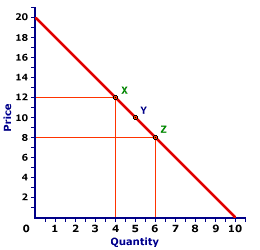Thursday  November 30, 2023
 AmosWEB means Economics with a Touch of Whimsy!AGGREGATE EXPENDITURE DETERMINANTS: An assortment of ceteris paribus factors that affect aggregate expenditures, but which are assumed constant when the aggregate expenditure line is constructed. Changes in any of the aggregate expenditures determinants cause the aggregate expenditure line to shift. While a wide variety of specific ceteris paribus factors can cause the aggregate expenditure line to shift, it's usually most convenient to group them into the four, broad expenditure categories -- consumption, investment, government purchases, and net exports. The reason is that changes in these expenditures are the direct cause of shifts in the aggregate expenditure line. If any determinant affects aggregate expenditures it MUST affect one of these four expenditures.ARC ELASTICITY:

The average elasticity for discrete changes in two variables. The distinguishing characteristic of arc elasticity is that percentage changes are calculated based on the average of initial and ending values of each variable, rather than initial values. Arc elasticity is generally calculated using the midpoint elasticity formula. The contrast to arc elasticity is point elasticity. For infinitesimally small changes in two variables, arc elasticity is the same as point elasticity.
Arc elasticity is best considered the average elasticity over a range of values for a relation. Like any average, some values within the range are likely to be greater and some less. However, it provides a quick approximation of elasticity when more precise and sophisticated calculation techniques are not possible.

### Working Through an Example

A Standard Demand CurveThe demand curve displayed to the right can be used to illustrate the measurement of arc elasticity using the midpoint elasticity formula. If the price declines from \$12 to \$8, the quantity demanded increases from 4 to 6, from point X to point Z. Using this midpoint formula (with price designated as P and quantity designated as Q) average price elasticity of demand is:
 midpointelasticity = (Q[Z] - Q[X])(Q[Z] + Q[X])/2 ÷ (P[Z] - P[X])(P[Z] + P[X])/2

 midpointelasticity = (6 - 4)(6 + 4)/2 ÷ (8 - 12)(8 + 12)/2 = (2)(5) ÷ (-4)(10)

 midpointelasticity = 0.4 ÷ -0.4 = -1

Ignoring the minus sign, the price elasticity of demand over this segment of the demand curve from X to Z is 1.0.

### An Average Value

This value of 1.0 is actually an average for the entire range between points X and Z. Precise estimates of point elasticity shows that the elasticity is 0.67 at point X and 1.5 at point Z. Moreover, the elasticity is different at each point on a straight line demand curve such as this one. The only point in which the elasticity is exactly equal to 1.0 is at point Y, the midpoint between X and Z.

This last observation is worth emphasizing. The midpoint elasticity formula effectively estimates the point elasticity at the very midpoint of the overall segment. This means that the elasticity of any point on a demand curve (point elasticity) can be obtained by calculating the arc elasticity with the midpoint elasticity formula such that the desired point is dead center in the middle, the midpoint of the arc.

 <= AMERICAN ECONOMIC ASSOCIATION ASSUMPTION =>Recommended Citation:

ARC ELASTICITY, AmosWEB Encyclonomic WEB*pedia, http://www.AmosWEB.com, AmosWEB LLC, 2000-2023. [Accessed: November 30, 2023].

Check Out These Related Terms...

Or For A Little Background...

And For Further Study...
Search Again?PINK FADFLY[What's This?] Today, you are likely to spend a great deal of time strolling through a department store hoping to buy either a coffee cup commemorating the 2000 Olympics or a birthday gift for your grandmother. Be on the lookout for neighborhood pets, especially belligerent parrots.Your Complete ScopeWoodrow Wilson's portrait adorned the \$100,000 bill that was removed from circulation in 1929. Woodrow Wilson was removed from circulation in 1924."Consult not your fears, but your hopes and your dreams. Think not about your frustrations, but about your unfulfilled potential. Concern yourself not with what you tried and failed in, but with what it is still possible for you to do. "-- Pope John XXIIIJPUBEJournal of Public EconomicsA PEDestrian's Guide Xtra CreditTell us what you think about AmosWEB. Like what you see? Have suggestions for improvements? Let us know. Click the User Feedback link.| | | | | | | | | | |
| | | |

Thanks for visiting AmosWEB